Courses

# Test: Magnetic & Electric Properties Of Solids & Semiconductors

## 24 Questions MCQ Test Chemistry for JEE | Test: Magnetic & Electric Properties Of Solids & Semiconductors

Description
This mock test of Test: Magnetic & Electric Properties Of Solids & Semiconductors for Class 12 helps you for every Class 12 entrance exam. This contains 24 Multiple Choice Questions for Class 12 Test: Magnetic & Electric Properties Of Solids & Semiconductors (mcq) to study with solutions a complete question bank. The solved questions answers in this Test: Magnetic & Electric Properties Of Solids & Semiconductors quiz give you a good mix of easy questions and tough questions. Class 12 students definitely take this Test: Magnetic & Electric Properties Of Solids & Semiconductors exercise for a better result in the exam. You can find other Test: Magnetic & Electric Properties Of Solids & Semiconductors extra questions, long questions & short questions for Class 12 on EduRev as well by searching above.
QUESTION: 1

### Only One Option Correct Type This section contains 7 multiple choice questions. Each question has four choices (a), (b), (c) and (d), out of which ONLY ONE is correct. Consider the following species. I. Mg(s) II. TiO(s) III. I2(s) IV. H2O (s) Q. Which of the above solids is/are electrical conductor?

Solution:

I. Mg (metal) conducts electricity in solid state as well as in molten state due to available free valence electrons.

II. TiO behaves like metals and is a good conductor of electricity.

III. I2 is a bad conductor.

IV. H2O (ice) is a bad conductor.

QUESTION: 2

### Which kind of defects are introduced by doping?

Solution:

(a) When smaller cation migrates to interstitial site, it is called dislocation defect. It is also called Frenkel defect.

(b) When equal number of cation and anion are missing or one cation (A+) is missing and charge is balanced by A2+ , then this is called Schottky defect.

(c) as in (a)

(d) Electronic defects (n-type, p -type) is due to doping of impurity.

QUESTION: 3

### Silicon doped with electron-rich impurity forms

Solution:

(b)

(a) When Si is doped with electron-deficient impurity -(p -type)

(b) When Si is doped with electron-rich impurity-(n-type)

(c) In case of semiconductors, the gap between the valence band and conduction band is small. Some electrons may Jump to conduction band and thus, conduction is observed. Semiconductors of this type are called intrinsic semiconductors.

(d) Insulators are non-conducting with high energy gap.

QUESTION: 4

Select the correct matching.

Solution:

(c)

When polar crystal is subjected to a mechanical stress, electricity is produced. It is called piezoelectricity.

Piezoelectric crystals with permanent dipoles (domains aligned in ! same direction) are said to have ferroelectricity as in (a) and with : zero dipoles (domains aligned equally in opposite direction) are said to have anti-ferroelectricity as in (b).

QUESTION: 5

Which of the following oxides behaves as conductor or insulator depending upon temperature?

Solution:

Metals are good conductors of electricity and have conductivities in the order 107(Ωm-1) or 105(Ωm-1).
Insulator: Solids having range of conductivity from 10-20 to 10-10 ohm-1 m-1 are considered as insulators.
Semiconductor: Solids having intermediate range of conductivity, i.e. from 10-6 to 104 ohm-1 m-1 are called semiconductors.

QUESTION: 6

How many energy levels are present in 3s conduction band of a single crystal of sodium weighing 26.8 mg?

Solution: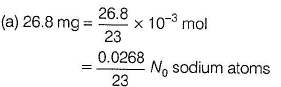Electrons in 3 s orbital in one sodium atom (conduction band) = 1

Thus, total conduction bands (energy levels =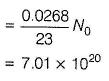QUESTION: 7

In which of the following oxides conducting or insulating property of is dependent on temperature?

Solution:

Ti2O3, conducting below 41OK and insulating at about 150 K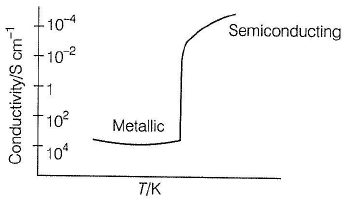*Multiple options can be correct
QUESTION: 8

One or More than One Options Correct Type
This section contains 5 multiple type questions. Each question has 4 choices (a), (b), (c) and (d), out of which ONE or MORE THAN ONE are correct.

Select the correct statement(s) about the movement of electrons and holes in a p-type semiconductor when an electric field is applied.

Solution:

(a,b) When Si or Ge is doped with a group 13 elements (B, Al, Ga) then, one valence electron of Si makes a hole or vacancy. When electric field is applied, electrons move towards positively charged plate through electronic holes. It would appear that electronic holes are positively charged and are moving towards negatively charged plate.

*Multiple options can be correct
QUESTION: 9

Select the correct statement(s).

Solution:

(a,b,c,d)

Si ( 1 4 ) - [Ne] 3s2 3p2 (group 14 element having four valence electrons). Thus, each atom of Si is covalently bonded to four neighbouring atoms.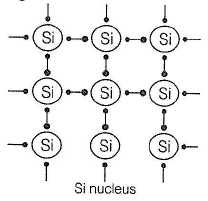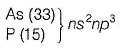3 (five valence electrons

Group 15 elements Four electrons are involved in bonding with | four electrons of Si and one remaining electron becomes I delocalised and increases the conductivity of Si. Thus, increase in I the conductivity is due to doped negatively charged electron. This is called n-type semiconductor.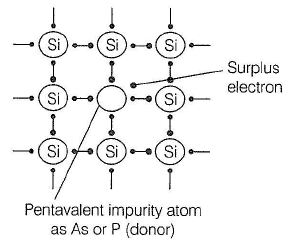Group 13 elements

[B, Al, Ga] ns2np1 In this case, B, Al or Ga is short of one electron (as compared to Si). This is called electron hole or electron vacancy. Under the influence of electric field, electron hole moves towards the positively charged plate and is called p -type semiconductor.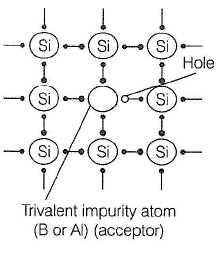QUESTION: 10

Which of the following oxides behaves as conductor or insulator depending upon temperature?

Solution:

The correct answer is option C
TiO3​ behaves as conductor or insulator depending on temperature because of a variation of energy gap between valence band and conduction band with the variation of temperature.

*Multiple options can be correct
QUESTION: 11

Select the correct statement(s).

Solution: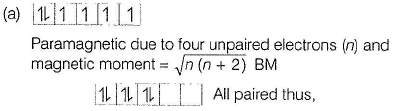n = 0, Magnetic moment = 0

Thus, (a) is correct.

(b) Paramagnetic substances are magnetised in a magnetic field in the same direction hence, weakly attracted thus, (b) is correct.

(c) Ferromagnetic substances can be magnetised permanently, thus, given statement is incorrect.

(d) Substances like MnO showing anti-ferromagnetism have domain structure similar to ferromagnetic substances, but their domains are oppositely oriented and cancel out each other's magnetic moment.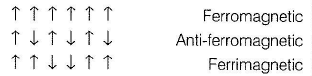*Multiple options can be correct
QUESTION: 12

Germanium has been doped with impurity as shown in I, II, III, IV. Select the correct statement(s).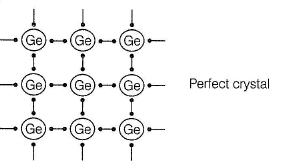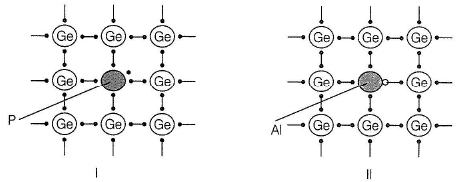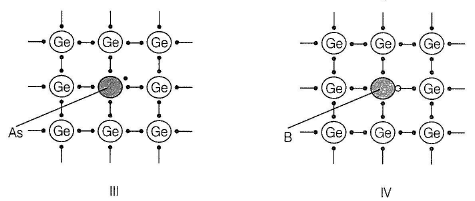Solution:

(a,b)

I and III have been doped with pentavalent impurity (P and As). There is one surplus electron and this results in the formation of n-type semiconductor. Surplus electron is responsible for conduction. II and IV have been doped with trivalent impurity (B and Al).
There is one electron short for bonding with Ge, hence a hole is formed. Hole moves through the electric field. This is p-type semiconductor.

QUESTION: 13

Direction (Q. Nos. 13-17) This section is based on Statement I and Statement II. Select the correct answer from the codes given below.

Q.

Statement I : Graphite is a good conductor of electricity.
Statement II : In graphite, carbon atom is sp2 hybridised.

Solution:

. (b)

Graphite has four valence electron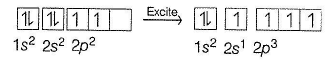Three electrons are involved in covalent bonding with neighbouring atoms giving it a hexagonal type packing (layers) such that one layer can slide over the other. The fourth electron makes the graphite conducting. Hexagonal sheets are with sp2- hybridised carbon.
Thus, both Statements I and II are correct but Statement II is not the correct explanation of Statement I.

QUESTION: 14

Statement I : A ferromagnetic substance becomes a permanent magnet, when it is placed in a magnetic field.

Statement II : All the domains get oriented in the direction of magnetic field.

Solution:

(a)

In solid state, metal ions of ferromagnetic substances are grouped together into small regions called domains. Each domain acts as a tiny magnet. In an unmagnetised ferromagnetic substance, domains are randomly oriented and their magnetic moments are cancelled. In a magnetic field, all the domains are oriented in the direction of the magnetic field producing a strong magnetic effect and magnetism persists permanently even after magnetic field is removed.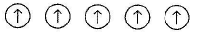Thus, both Statements I and II are correct and Statement II is the correct explanation of Statement I

QUESTION: 15

Statement I : In the case of anti-ferrom agnetic substances, magnetic moment is zero.

Statement II : Domains get oriented in the direction of applied magnetic field.

Solution:

(c)

Anti-ferrom agnetic substances (as MnO) have following domain arrangements

following domain arrangements.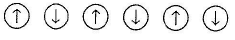These domains are oppositely oriented and cancel out each other's magnetic moment and making it zero (even in the absence of magnetic field).
Thus, Statement I is correct and Statement II is incorrect.

QUESTION: 16

Statement I : The electrical conductivity of a metal decreases as temperature increases.

Statement II : This impedes the flow of electrons, when an electric field is applied.

Solution:

(b)

Increase in temperature causes thermal agitation of metal ions and thus, electrical conductivity is decreased. Thus, Statement I is correct. In the presence of electric field, flow of electrons is impeded. Thus, Statement II is correct but is not the correct explanation of Statement I.

QUESTION: 17

Statement I : Electrical conductivity of semiconductors increases with rise in temperature.

Statement II : There is a small energy gap between conduction band and valence band.

Solution:

(b) Sem iconductors have small energy g ap between conduction band and valence band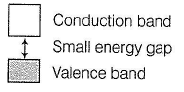On increasing temperature, electron from valence band can jump to conduction band and thus, electrical conductivity is increased. Thus, both Statements I and II are correct but Statement II is not the correct explanation of Statement I.

QUESTION: 18

Direction (Q . No. 18) Choices for the correct combination of elements from Column I and Column II are given as options (a), (b), (c) and (d), out of which one is correct.

Q.

Match the items in Column I with the terms in Column II and select the answer from the codes given: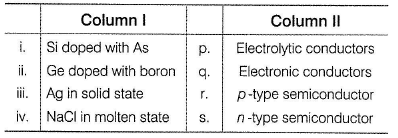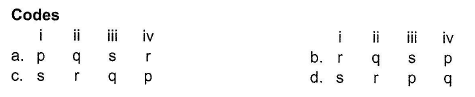Solution:

(i) Si doped with As has one extra electron for conduction, thus n-type semiconductor.

(ii) Ge doped with B has short of one electron thus, a hole is formed, thus a p-type semiconductor.

(iii) Metals have sea of electrons responsible for electronic conduction.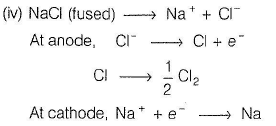Electron flow is due to electrolysis. Thus, electrolytic conduction.
Thus, (i) (s),

(ii) -> (r),

(iii) -» (q),

(iv) (p).

QUESTION: 19

Direction (Q. Nos. 19 and 20) This section contains 1 paragraph, which describing theory, experiments, data, etc. Two questions related to the paragraph have been given. Each question has only one correct answer among the four given options (a), (b), (c) and (d).

Passage

The following pictures show the electrons population of the composite s-d bands for three transition metals :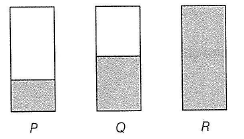Metals with the highest and lowest melting points respectively are

Solution:

P indicates that bonding molecular orbitals are empty.

Q indicates that bonding molecular orbitals are filled.

R indicates that bonding and antibonding molecular orbitals are filled.

Highest melting point is for the metal having filled bonding molecular orbitals (they have maximum bond order). Lowest melting point is for the metal having filled bonding and anti-bonding molecular orbitals thus, bond order is zero. Increasing order of hardness of the metals is R < P< Q

Hardness increases with increasing bond order.

QUESTION: 20

Passage

The following pictures show the electrons population of the composite s-d bands for three transition metals :Hardness of the metals in the increasing order is

Solution:

P indicates that bonding molecular orbitals are empty.

Q indicates that bonding molecular orbitals are filled.

R indicates that bonding and antibonding molecular orbitals are filled.

Highest melting point is for the metal having filled bonding molecular orbitals (they have maximum bond order). Lowest melting point is for the metal having filled bonding and anti-bonding molecular orbitals thus, bond order is zero. Increasing order of hardness of the metals is

R < P< Q

Hardness increases with increasing bond order.

*Answer can only contain numeric values
QUESTION: 21

Direction (Q. Nos. 21-24) This section contains 4 questions. When worked out will result in an integer from 0 to 9 (both inclusive).

Q.

How many of the following species show paramagnetism?

TiO, VO, V2O5 , NaCl, C6H6 , H2O, CH3COCH3, CuCl

Solution:

(5)

Species with atieast one or more unpaired electron(s) are attracted by the magnetic field and is said to be paramagnetic.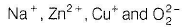No unpaired electron hence, diamagnetic.

O- Two unpaired electrons in anti bonding molecular orbitals.

Fe2+ - Four unpaired electrons in 3d-orbital.

Cr3+ - Three unpaired electrons in 3c/-orbital.

Cu+ - One unpaired electron in 3d-orbital.

O-2 One unpaired electron in antibonding molecular orbitals.

*Answer can only contain numeric values
QUESTION: 22

Magnetic moment of a compound showing paramagnetism is 54.842 x 10-24 Am2 (Bohr magneton). How many unpaired electrons are there in the compound?

Solution: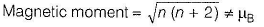where, n = number of unpaired electrons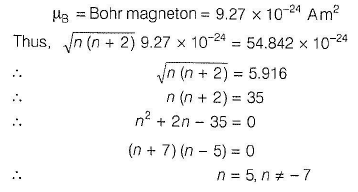QUESTION: 23

How many of the following are diamagnetic in nature?

Solution:

Diamagnetic substance have all paired electron.
(a) [Fe(CN)6​]3−Oxidation state of Fe=+3
It has one unpaired electron and is paramagnetic.
(b) NiCl42−​ - It has two unpaired electrons and is paramagnetic
(c) [Ni(CO)4​] - It has no unpaired electron and is diamagnetic
(d) [MnCl4​]2− - It is paramagnetic as it has five unpaired electrons.

*Answer can only contain numeric values
QUESTION: 24

How many of the following are antiferromagnetic substances?

MnO, MnO2, Mn2O, FeO, Fe2O3, NiO ,Cr2O3, CoO, Co3O4 , H20, NaCl, C6H6

Solution:

(9) Antiferromagnetism arises when net dipole alignment is zero due to equal and opposite alignment. This is observed in

MnO, MnO2, Mn2O, FeO, Fe2O3, NiO ,Cr2O3, CoO, Co3O4.